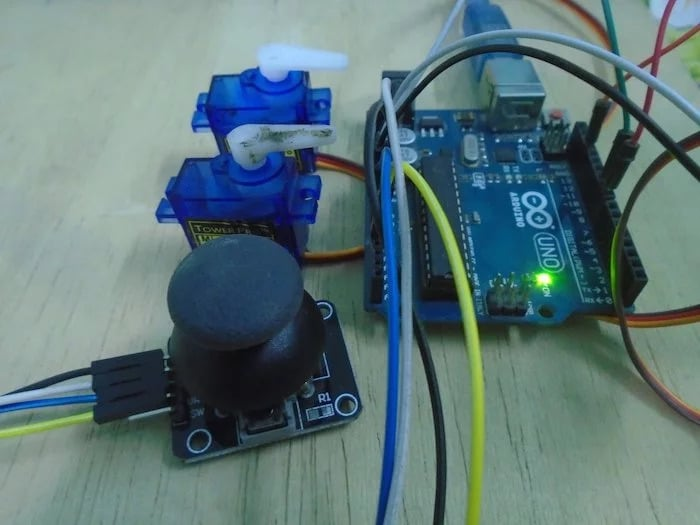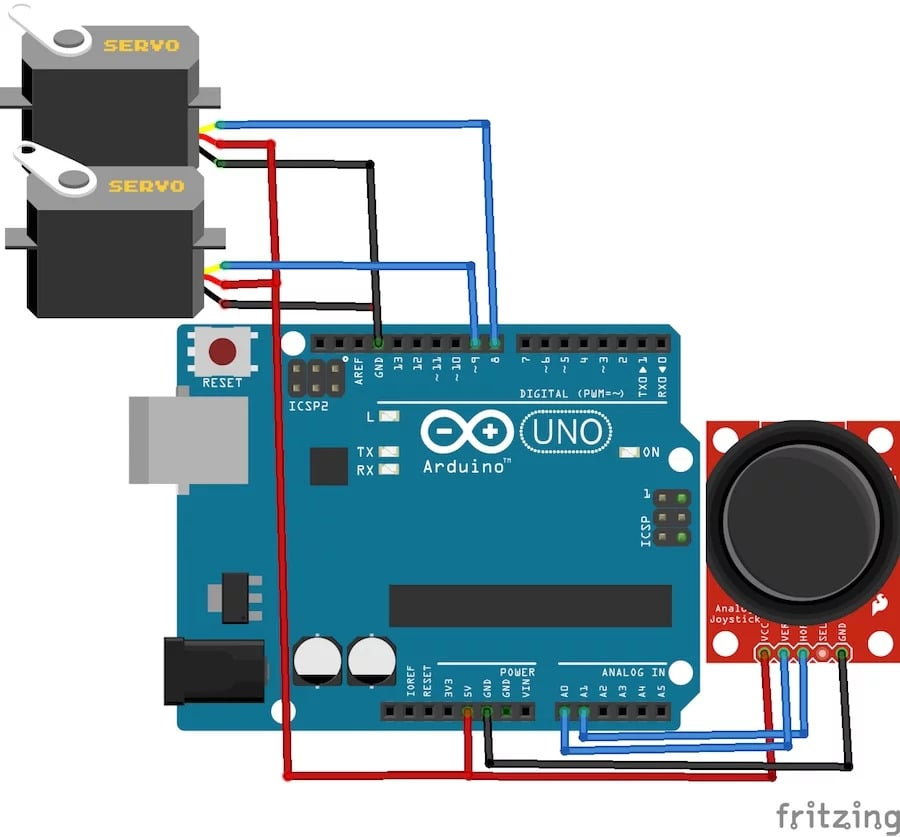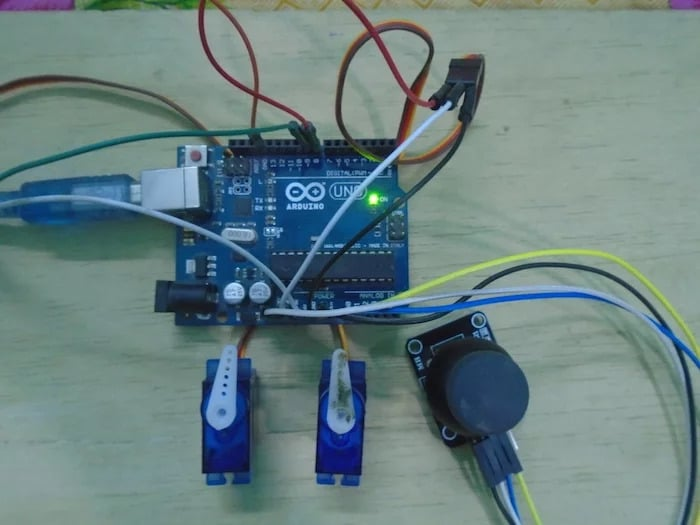Arduino

# How to Control Servo Motors With an Arduino and JoystickMarch 22, 2018 by A .
ShareControl servo motors with a joystick module connected to an Arduino.### Circuit Diagram

The hardware part of this project is very easy to make. First, connect the joystick module with the Arduino. The connections for the joystick module and the Arduino are as follows:

• Connect the VCC on the joystick module with the 5V pin on the Arduino
• Connect the GND pin on the joystick module with the GND on the Arduino
• Connect the VER pin on the joystick module with the A0 on the Arduino
• Connect the HOR pin on the joystick module with the A1 on the Arduino
After that, connect the servo motors with the Arduino. The connections for servo motors with Arduino are as follows:

• Connect the black wire on both the servo motors with the GND on the Arduino
• Connect the red wire on both the servo motors with the 5V pin on the Arduino
• Connect the yellow wire on the first motor with pin 8 on the Arduino
• Connect the yellow wire on the second motor with pin 9 on the Arduino### How Does It Work?

When the joystick module moves in the horizontal or in the vertical direction, it gives us values from 0 to 1023. So we can apply a condition in the code that if the value is less than 300 or greater than 700, then the servos will move.

When the joystick is moved in the horizontal direction, the first servo will move towards right or left and upon moving the joystick in the vertical direction, the second servo will move towards the right or left.

### Arduino Code

``````#include
Servo servo1;
Servo servo2;
int x_key = A1;
int y_key = A0;
int x_pos;
int y_pos;
int servo1_pin = 8;
int servo2_pin = 9;
int initial_position = 90;
int initial_position1 = 90;

void setup ( ) {
Serial.begin (9600) ;
servo1.attach (servo1_pin ) ;
servo2.attach (servo2_pin ) ;
servo1.write (initial_position);
servo2.write (initial_position1);
pinMode (x_key, INPUT) ;
pinMode (y_key, INPUT) ;
}

void loop ( ) {

if (x_pos < 300){
if (initial_position < 10) { } else{ initial_position = initial_position - 20; servo1.write ( initial_position ) ; delay (100) ; } } if (x_pos > 700){
if (initial_position > 180)
{
}
else{
initial_position = initial_position + 20;
servo1.write ( initial_position ) ;
delay (100) ;
}
}

if (y_pos < 300){
if (initial_position1 < 10) { } else{ initial_position1 = initial_position1 - 20; servo2.write ( initial_position1 ) ; delay (100) ; } } if (y_pos > 700){
if (initial_position1 > 180)
{
}
else{
initial_position1 = initial_position1 + 20;
servo2.write ( initial_position1 ) ;
delay (100) ;
}
}
}``````

### Code Explanation

First of all, we included the library for the servo motor which will help us with making the code easier. Then, we initialized two variables, one for each of the two servo motors which will help us in using the library functions.

``````#include
Servo servo1;
Servo servo2;``````

Then, we initialized the pins where we have connected the vertical and horizontal pins on the joystick module and also the signal pins on the servos.

``````int x_key = A1;
int y_key = A0;
int x_pos;
int y_pos;
int servo1_pin = 8;
int servo2_pin = 9;
int initial_position = 90;
int initial_position1 = 90;``````

Then we tell the Arduino where we have connected the servo pins and also moved the servo motors at the initial position, which is 90 degrees. After that, we declared both the vertical and horizontal pins on joystick module as the input pins.

``````servo1.attach (servo1_pin ) ;
servo2.attach (servo2_pin ) ;
servo1.write (initial_position);
servo2.write (initial_position1);
pinMode (x_key, INPUT) ;
pinMode (y_key, INPUT) ;``````

In the loop function, we read the values for the horizontal and the vertical position from the joystick module and saved these in the variables. Then we applied a condition that if the value for the horizontal position is less than 300, then the first servo will move towards the right.

``````x_pos = analogRead (x_key) ;
if (x_pos < 300){
if (initial_position < 10)
{
}
else{
initial_position = initial_position - 20;
servo1.write ( initial_position ) ;
delay (100) ;
}
}``````

If the value for the horizontal position is greater than 700, then the servo will move towards the left. Similarly for the vertical position of the joystick module, if the value is less than 300, then the second servo will move towards the left, and if the value is greater than 700, then the second servo will move towards the right.

``````if (x_pos > 700){
if (initial_position > 180)
{
}
else{
initial_position = initial_position + 20;
servo1.write ( initial_position ) ;
delay (100) ;
}
}``````### AuthorA .

For custom projects, hire me at https://www.freelancer.pk/u/Muhammadaqibdutt